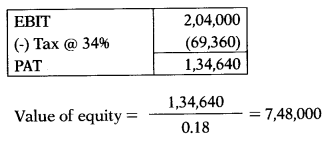# Valuation, Principles & Framework – Corporate and Management Accounting MCQ

Going through the Valuation, Principles & Framework – Corporate and Management Accounting CS Executive MCQ Questions with Answers you can quickly revise the concepts.

## Valuation, Principles & Framework – Corporate and Management Accounting MCQs

Question 1.
Which of the following statement is incorrect regarding ‘Business Valuation?
(A) It is an examination conducted towards rendering an estimate or opinion as to the fair market value of a business interest at a given point in time.
(B) Valuation is an art rather than an exact science.
(C) Business valuation is a precise science.
(D) Valuation estimates and opinions are generally stated as a range of values.
(C) Business valuation is a precise science.Question 2.
Assertion (A):
Valuation can be used as a very effective business tool by management for better decision making throughout the life of the enterprise.
Reason (Re-valuations are needed for many reasons such as investment analysis, capital budgeting, merger and acquisition transactions, financial reporting, determination of tax liability and in litigation.
Select the correct answer from the options given below:
(A) A is true but R is false
(B) A is false but R is true
(C) Both A and R true but R is not correct explanation of A
(D) Both A and R true and R is correct explanation of A
(D) Both A and R true and R is correct explanation of A

Question 3.
A business valuation is an examination conducted towards rendering as to the fair market value of a business interest at a given point in time.
(A) An estimate
(B) An Opinion
(C) An estimate or opinion
(D) Ruling or judgment
(C) An estimate or opinion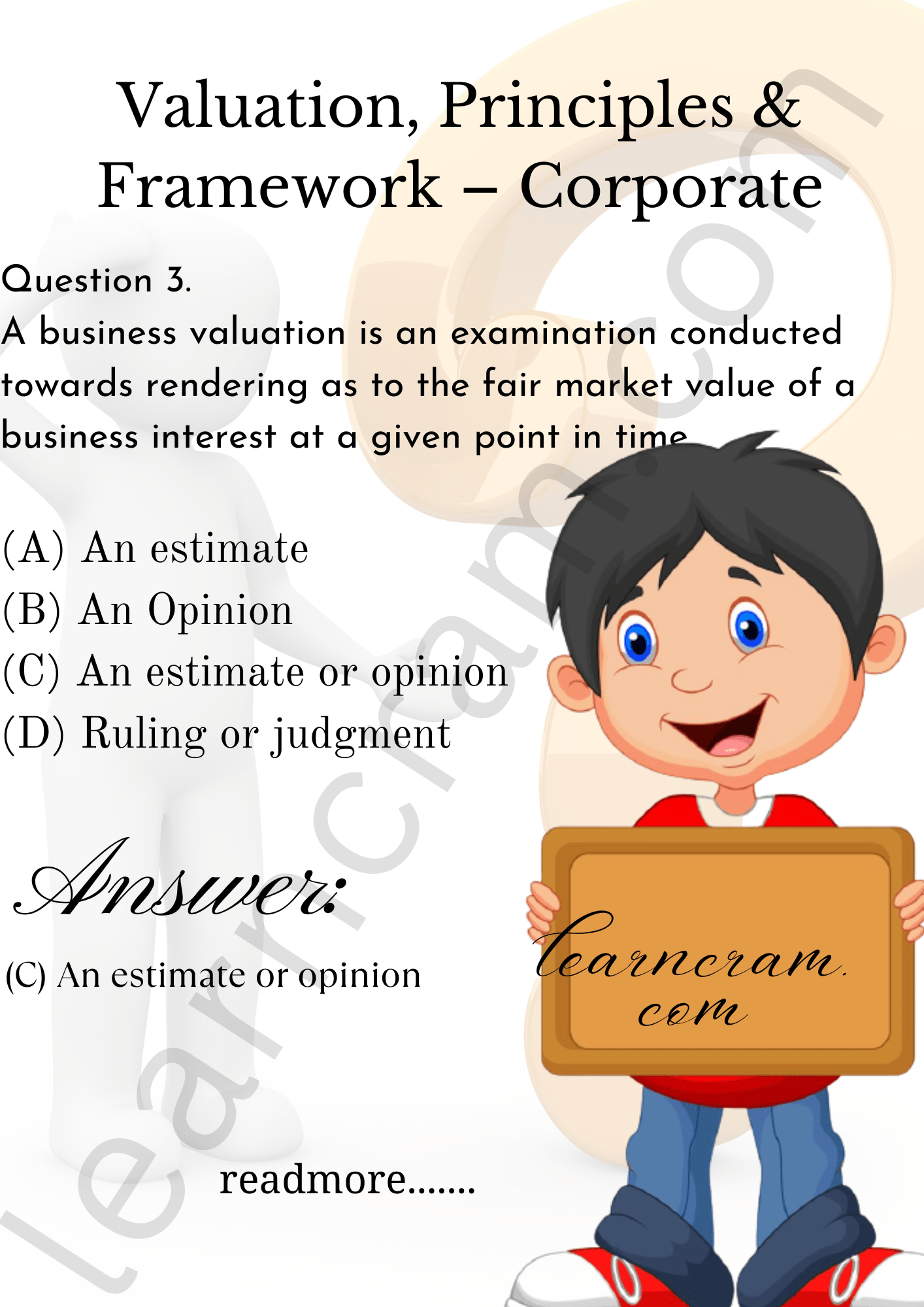Question 4.
Which of the following item is not deducted while calculating Capital Employed as per ‘Long Term Funds Approach?
(A) Outstanding & accrued interest
(B) Tax provisions
(C) Debentures
(D) Non-current liabilities
(C) Debentures

Question 5.
Like accounting, valuation is –
(A) Method
(B) An exact science
(C) An art rather than an exact science
(D) None of the above
(C) An art rather than an exact science

Question 6.
Which of the following item will not be deducted while calculating shareholders funds as per Net Asset value Method?
(A) Debentures
(B) Liabilities not provided in account
(C) Dividend
(D) Outstanding expenses
(C) Dividend

Question 7.
As per Net Asset value Method while calculating capital employed debtors are taken at
(A) Book value
(B) Realizable value
(C) Present value
(D) Value in use
(B) Realizable value

Question 8.
Companies in merger need valuation of business as a going concern to settle the:
(A) Assets
(B) Liabilities
(C) Purchase consideration
(D) Deferred tax
(C) Purchase consideration

Question 9.
Net asset value per share is also known as –
(A) Internal value per share
(B) Intrinsic value per share
(C) Economic value per share
(D) Recoverable value per share
(B) Intrinsic value per share

Question 10.
A number of different measurement bases are employed to different degrees and in varying combinations in valuation of different assets in different areas of application. You are required to state which of the following base is not used in business valuation?
(A) Historical Cost
(B) Current Cost
(C) Realizable Value
(D) Notional cost
(D) Notional cost

Question 11.
Which of the following is deducted while calculating net assets available to equity shareholders?
(A) Proposed preference dividend
(B) Share suspense account
(C) Know-how
(A) Proposed preference dividend

Question 12.
As per historical cost concept -…………
(A) Assets are carried at the amount of cash or cash equivalents that would have to be paid if the same or an equivalent asset were acquired currently.
(B) Assets are carried at the amount of cash or cash equivalents that could currently be obtained by selling the asset in an orderly disposal.
(C) Assets are recorded at the amount of cash or cash equivalents paid or the fair value of the other consideration given to acquire them at the time of their acquisition.
(D) Assets are carried at the present value of the future net cash inflows that the item is expected to generate in the normal course of business.
(C) Assets are recorded at the amount of cash or cash equivalents paid or the fair value of the other consideration given to acquire them at the time of their acquisition.

Question 13.
Market based methods of valuation should not be adopted when -………..
(A) When business is too small
(B) When assets are less than liabilities of the business
(C) In case of significant and unusual fluctuations in market price
(D) It is difficult to estimate the realizable value in case of going concern.
(C) In case of significant and unusual fluctuations in market price

Question 14.
………….. is same as the Realizable (settlement) value. This is the value (net of expenses) that can be realized by disposing off the assets in an orderly manner.
(A) Present Value
(B) Historical Cost
(C) Realizable (Settlement) Value
(D) Liquidation Value
(C) Realizable (Settlement) Value

Question 15.
Market value method is generally the most preferred method in case of -…………
(A) Frequently traded shares of companies listed on stock exchanges having nationwide trading
(B) Valuation of a division of a company
(C) Where the share are not listed or are thinly traded
(D) Where there is an intention to liquidate it and to realize the assets and distribute the net proceeds.
(A) Frequently traded shares of companies listed on stock exchanges having nationwide trading

Question 16.
Discounted cash flow valuation is based upon -………….
(A) Expected future discount that likely to be earned.
(B) Real worth of the business
(C) Expected future cash flows and discount rates.
(D) Earning capacity of the company
(C) Expected future cash flows and discount rates.

Question 17.
Net Realizable Value means –
(B) Same value as the present value.
(C) Value net of expenses.
(D) Higher of the net selling price and value in use.
(C) Value net of expenses.

Question 18.
Statement I:
Net Asset Method can be fairly used to value shares when the firm is liquidated.
Statement II:
This method does not give any weight to earning capacity of the company.
Select the correct answer from the options given below:
(A) Statement I is correct but Statement II is incorrect
(B) Statement I is incorrect but Statement II is correct
(C) Both Statement I and Statement II are incorrect
(D) Both Statement I and Statement II are correct
(D) Both Statement I and Statement II are correct

Question 19.
Which of the following is normally used as discounting factor under the discounted cash flow valuation?
(A) Cost of equity
(B) Cost of debt
(C) Annuity factor
(D) Overall cost of capital
(D) Overall cost of capital

Question 20.
Present value means –
(A) Value in use
(B) Value of future cash flows
(C) Value calculated using IRR
(B) Value of future cash flows

Question 21.
Which of the following is required to be taken into consideration while valuing equity shares of the company?
(A) Size of the block of shares
(B) Restricted transferability aspect
(C) Dividends
(D) All of the above
(D) All of the above

Question 22.
Match the following:
List-I — List-II
A. Present Value — 1. Lower of the replacement value & recoverable value
B. Liquidation value — 2. Higher of the net selling price and value in use
C. Deprival Value — 3. Value of the future net cash inflows
D. Recoverable Value — 4. Value net of expenses
Select the correct answer from the options given below:(B)

Question 23.
Which of the following is correct formula for Capitalization of Earning Method?
(A) ‘Net Operating Income’ divided by ‘Capitalization Rate’
(B) ‘Net Profit’ divided by ‘Discount Rate’
(C) ‘Net Operating Income’ divided by ‘Growth Rate’
(D) ‘Dividend Per Share’ divided by ‘Annuity Rate’ multiplied by total number of shares
(A) ‘Net Operating Income’ divided by ‘Capitalization Rate’

Question 24.
In which of the following cases valuation is essential?
(A) Conversion of debt instruments into shares.
(B) On directions of Tribunal or Authority or Arbitration Tribunals.
(C) When issuing shares to public either through an Initial Public Offer or by offer for sale.
(D) Valuation is always depends on fact that who is valuing what.
(D) Valuation is always depends on fact that who is valuing what.

Question 25.
Which of the following is another name for the required return on a stock?
(A) Discount rate
(B) Dividend payout ratio
(C) Retention ratio
(D) Value
(A) Discount rate

Question 26.
Which of the following is not a general principle involved in business valuation?
(A) Value is determined at a specfic point in time.
(B) Value is prospective.
(C) Value is influenced by liquidity.
(D) Valuation is always depends on fact that who is valuing what.
(D) Valuation is always depends on fact that who is valuing what.

Question 27.
Which of the following is equal to the present value of all cash proceeds received by a stock investor?
(A) Value
(B) Retention ratio
(C) Dividend payout ratio
(D) Discount rate.
(A) Value

Question 28.
Which of the following do financial analysts consider least important when assessing the long-run economic and financial outlook of a company?
(A) Expected return on equity
(B) Prospects of the relevant industry
(C) Expected changes in EPS
(D) General economic conditions
(D) General economic conditions

Question 29.
Provisions relating to ‘valuation by registered valuers’ are contained in -…………
(A) Section 247 of the Companies Act, 2013
(B) Section 242A of the Income-tax Act, 1961
(C) Section 347 of the Companies Act, 2013
(D) Section 240AB of the Income-tax Act, 1961
(A) Section 247 of the Companies Act, 2013

Question 30.
Which of the following is NOT a method of business valuation?
(A) Asset based
(B) Earnings based
(C) Market based
(D) Equity based
(D) Equity based

Question 31.
Analysts commonly consider all of the following to be indicators that the market is overvalued except…………..
(A) High average P/E ratio
(B) High average price-to-book ratio
(C) High average dividend yield
(D) All of the above
(C) High average dividend yield

Question 32.
Which of the following best describes the replacement value of a business?
(A) Value if sold off piece-meal
(B) Value to replace assets with new
(C) Cost of setting up an equivalent venture
(D) Net present value of current operations
(B) Value to replace assets with new

Question 33.
As per Section 247 of the Companies Act, 2013, where a valuation is required to be made in respect of any property, stocks, shares, debentures, securities or goodwill or any other assets or net worth of a company or its liabilities, it shall be valued by a person having such qualifications and experience and registered as a valuer in such manner, on such terms and conditions as may be prescribed and appointed by the –
(A) Audit committee
(B) Board of Directors of the company.
(C) Board of Directors on recommendation of audit committee.
(D) Audit committee or in its absence by the Board of Directors of that company.
(D) Audit committee or in its absence by the Board of Directors of that company.

Question 34.
Which of the following best defines the market capitalization for a company’s shares?
(A) When a company is listed ie. goes ‘public’
(B) When a company issues new shares and thus increases its capital
(C) Current share price
(D) Share price X number of shares in issue
(D) Share price X number of shares in issue

Question 35.
Value of perpetual bond is calculated by:
(A) Interest divided by rate of cost of equity
(B) Fixed income on security divided by required rate of return
(C) Fixed income on security divided by growth rate
(D) Interest divided by rate of growth
(B) Fixed income on security divided by required rate of return

Question 36.
As per Section 247(3) of the Companies Act, 2013, if a valuer contravenes the provisions of this section or the rules made thereunder, the valuer shall be punishable with fine which shall not be less than but which may extend to …………
(A) ₹ 50,000; ₹ 1,00,000.
(B) ₹ 25,000;₹ 1,00,000.
(C) ₹ 75,000; ₹ 1,50,000.
(D) ₹ 25,000; ₹ 50,000.
(B) ₹ 25,000;₹ 1,00,000.

Question 37.
The value of ……….. is the present value of its par value for maturity years discounted at required rate of return.
(A) Irredeemable bond
(B) Perpetual bond
(C) Volatility of bond
(D) Deep discount bond
(D) Deep discount bond

Question 38.
Value of business as per Asset based valuation -…………
(A) Liquidation value of assets minus replacement value of liabilities
(B) Market value of assets minus present value of liabilities
(C) Current/realizable value assets minus current value liabilities
(D) Current assets minus current liabilities
(C) Current/realizable value assets minus current value liabilities

Question 39.
The rate of tax affects the –
(A) Cost of retained earning
(B) Cost of debt
(C) Cost of equity
(D) All of the above
(D) All of the above

Question 40.
Floatation costs are those expenses which are incurred while –
(A) issuing securities
(B) repayment of debts
(D) repayment of equity and debts
(A) issuing securities

Question 41.
From the following details calculate the net asset value:
Fixed assets 1,000 lakh (1,215 lakh)
Cash in hand 35 lakh
Current assets 440 lakh (470 lakh)
Current liabilities 55 lakh
Dividend payable 6 lakh
Figures in bracket indicates market prices of the assets/liabilities.
(A) 1,665 lakh
(B) 1,629 lakh
(C) 1,420 lakh
(D) 1,720 lakh
(A) 1,665 lakh
1,215 + 35 + 470-55 = 1,665

Question 42.
Following details are available for two Y Ltd. has 40 lakh Equity Shares of ₹ 75 paid-up.What is the intrinsic value per share for these companies?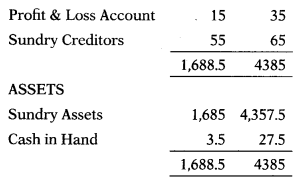X Ltd. has 9 lakh Equity Shares of 150 each, 135 paid-up.
Y Ltd. has 40 lakh Equity Shares of 75 paid-up.
What is the intrinsic value per share for these companies?
(A) ₹ 185.10 & ₹ 180 per share
(B) ₹ 118.50 & ₹ 102 per share
(C) ₹181.50 & ₹ 108 per share
(D) ₹ 185.10 & ₹ 102 per share
(C) ₹181.50 & ₹ 108 per share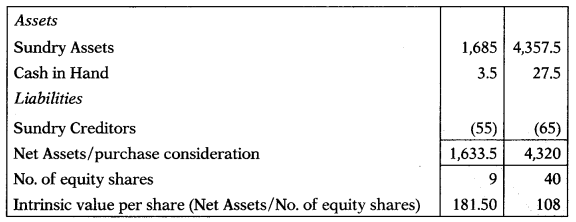Question 43.
Ramola Ltd. gives the following cash flows estimate:
2015: ₹ 2,000 lakhs
2016 to 2018: Compound growth rate 6.5%
2019 to 2022: Compound growth rate 9.5%
(A) ₹ 9,530.07 lakh
(B) ₹ 9,053.70 lakh
(C) ₹ 9,750.03 lakh
(D) ₹ 9,350.07 lakh
(D) ₹ 9,350.07 lakh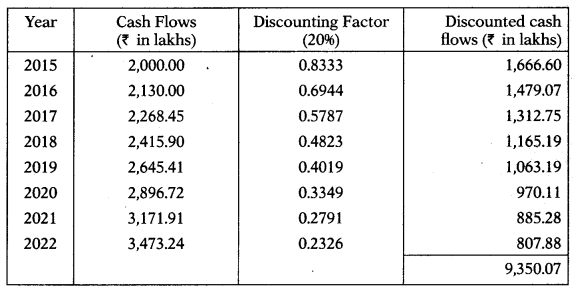Question 44.
If business is likely to generate cash flow as given below in next 5 years then what is the value of business as per discounted cash flow method.
Year 1: 120 lakh
Year 2: 160 lakh
Year 3: 200 lakh
Year 4: 280 lakh
Year 5:340 lakh
Use 20% discount factor.
(A) ₹ 598.53 lakh
(B) ₹ 589.53 lakh
(C) ₹ 578.35 lakh
(D) ₹ 553.98 lakh
(A) ₹ 598.53 lakhQuestion 45.
Balance sheets of FDL Ltd. and GCL Ltd. are as follows: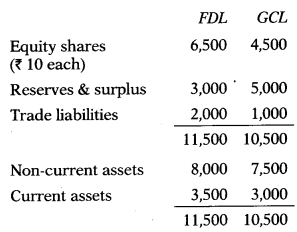Both companies were amalgamated and a new company WWL Ltd. was formed. Fixed assets of FDL were valued at ₹ 10,000 and that of GCL were valued at ₹ 9,000. WWL would issue the requisite number of equity shares of ₹ 10 each at 50% premium to discharge the claim of equity shareholders of FDL and GCL. How many shares of WWL should be issued to take over the business of the two merging companies?
(A) 1,200 shares
(B) 1,500 shares
(C) 1,334 shares
(D) 1,800 shares
(B) 1,500 shares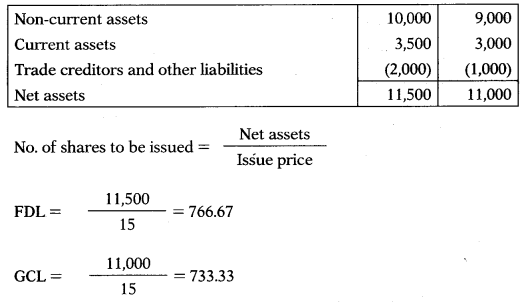Total shares to be issued = 766.67 + 733.33 = 1,500

Question 46.
The Wind Urja Ltd. (WUL) is a closely held unlisted company with financial details as under:Worldwide Wind Energy Ltd. is ready to takeover WUL by paying 35% premium over the market value of assets and liabilities as goodwill. Calculate the price which WWEL is ready to pay to shareholders of WUL.
(A) ₹ 7,535 lakhs
(B) ₹ 10,725 lakhs
(C) ₹ 10,172.25 lakhs
(D) ₹ 12,217.52 lakhs
(C) ₹ 10,172.25 lakhs

Question 47.
The shares of company are selling at ₹ 45 per share. The firm had paid dividend @ ₹ 4.5 per share last year. The estimated growth of the company is approximately 5% per year. Determine the cost of equity of the company.
(A) 15.5%
(B) 12.3%
(C) 11.4%
(D) 16.8%
(A) 15.5%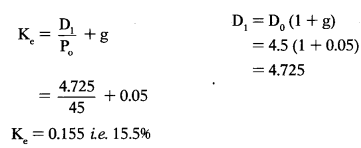Question 48.
The shares of company are selling at ₹ 20 per share. The firm had paid dividend @ ₹ 2 per share last year. The estimated growth of the company is approximately 8% per year. Required rate of return is 15.5%. Market value of equity shares as per dividend growth model will be:
(A) ₹ 32.4 per share
(B) ₹ 28.8 per share
(C) ₹ 25.5 per share
(D) ₹ 29.1 per share
(B) ₹ 28.8 per share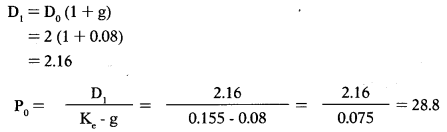Question 49.
ZPA Ltd. is foreseeing a growth rate of 12% p.a. in the next two years. The growth rate is likely to fall to 10% for the third year and forth year. After that growth rate is expected to stabilize at 8% p.a. If the last dividend (D0) paid was ₹ 1.5 per share and investor’s required rate of return is 16%, find out the intrinsic value per share of ZPA Ltd. as of date.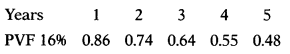(A) ₹ 22.33 per share
(B) ₹ 23.32 per share
(C) ₹ 33.22 per share
(D) ₹ 23.23 per share
(A) ₹ 22.33 per share

Question 50.
Balance Sheet of Smileheavy Ltd. as at 31.3.2019 reveals as under: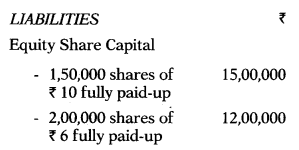Current value of Land & Buildings is ₹ 30,00,000, Furniture, Fixture & Fittings is ₹ 2,50,000. Inventory is valued at ₹ 9,11,000. Debtors are expected to realize 90% of their book value. You are informed that preference dividend has not been paid for the last 5 years. Calculate the intrinsic value of per equity shares having face value of ₹ 6 each fully paid-up by Net Assets Method.
(A) ₹ 9.037 per share
(B) ₹ 5.037 per share
(C) ₹ 6.42 per share
(D) ₹ 5.42 per share
(D) ₹ 5.42 per share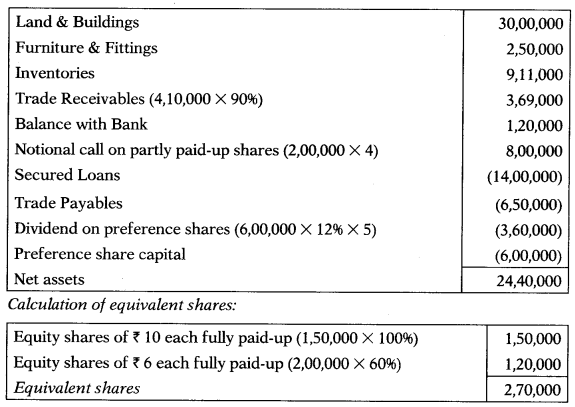Question 51.
A large chemical company has been expected to grow at 14% per year for next 4 years and then to grow indefinitely at the same rate as national economy ie. 5%. The required rate of return on equity share is 12%. Assume that company paid a dividend of ₹ 2 per share last year (D0 = 2). Determine the market price of the shares today.
(A) ₹ 42.60 per share
(B) ₹ 46.20 per share
(C) ₹ 40.62 per share
(D) ₹ 45.46 per share
(C) ₹ 40.62 per shareQuestion 52.
The required rate of return of investors is 15%. ABC Ltd. declared and paid annual dividend of ₹ 4 per share. It is expected to grow @ 20% for the next 2 years and 10% thereafter. Compute the price at which the shares should sell.
PVFactors: @ 15% for Year 1 = 0.8696 and Year 2= 0.7561.
(A) ₹ 108.80 per share
(B) ₹ 104.40 per share
(C) ₹ 110.10 per share
(D) ₹ 105.25 per share
(B) ₹ 104.40 per share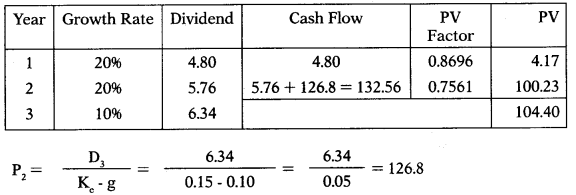Intrinsic value of ABC Ltd. is 104.40.

Question 53.
Anurag has invested in a share whose dividend is expected to grow @15% for 5 years and thereafter @ 5% till life of the company. Find out the value of the share, if current dividend is ₹ 4 per share and investors required rate of return is 6%.
(A) ₹ 656.93 per share
(B) ₹ 665.39 per share
(C) ₹ 666.99 per share
(D) ₹ 693.56 per share
(A) ₹ 656.93 per share

Question 54.
A bond has face value of ₹ 1,000 and coupon rate is 8%. Interest on bond is payable annually. Find the value of bond if the required rate of return is 6% and bond is perpetual bond.
(A) ₹ 1,333
(B) ₹ 1,667
(C) ₹ 1,445
(D) ₹ 1,222
(A) ₹ 1,333Question 55.
A bond has face value of ₹ 1,000 and coupon rate is 8%. Interest on bond is payable annually. Find the value of bond if the required rate of return is 10% and bond has maturity of 20 years.
(A) ₹ 681.12
(B) ₹ 849.21
(C) ₹ 830.12
(D) ₹ 765.48
(C) ₹ 830.12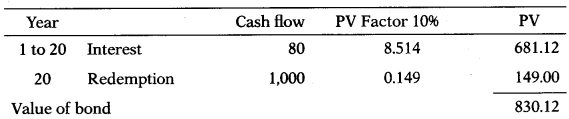Question 56.
ZPA Ltd. issued ₹ 1,000 optionally convertible debentures at a coupon rate of 10%. These debentures are convertible to 50 equity shares today. The shares are quoting ₹ 25 in stock market. Investor expects 8% return on his investment. Debentures are redeemable after 5 years. Will you suggest conversion?
(A) No, as fair value of debenture is ₹ 1,080.30 which is more than fair market value of shares
(B) No, as fair value of debenture is ₹ 1,170.60 which is less than fair market value of share
(C) Yes, as fair value of debenture is ₹ 1,080.30 which is less than fair market value of shares
(D) Yes, as fair value of debenture is ₹ 1,238.30 which is more than fair market value of shares
(C) Yes, as fair value of debenture is ₹ 1,080.30 which is less than fair market value of sharesMarket value of shares =50 × 25= 1,250
Analysis: Since market value of shares is more than fair value of debenture an investor is advised to convert debentures into equity shares.

Question 57.
Zensar Ltd. issued 5 year 12% Bond. Face value of the bond is ₹ 1,000 which is redeemable in 5 equal installments. What would be the current price of the bond if the YTM is 10%?
(A) ₹ 1,170.60
(B) ₹ 1,080.30
(C) ₹ 1,048.13
(D) ₹ 1,238.30
(C) ₹ 1,048.13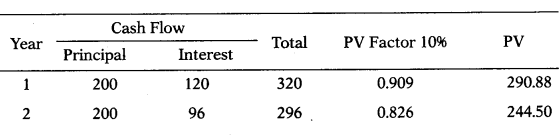Question 58.
What is value of deep discount bond issued by IDBI for a maturity period of 15 years and having a par value of ₹ 1,00,000 if the required rate of return is 12%?
(A) ₹ 12,000
(B) ₹ 15,400
(C) ₹ 22,600
(D) ₹ 18,300
(D) ₹ 18,300
The value of deep discount bond is the present value of ₹ 1,00,000 for 15 years discounted at 12%. The value of deep discount bond = 1,00,000 × 0.183 = 18,300

Question 59.
A Zero Coupon Bond (ZCB) was issued at a face value of ₹ 2,50,000 and has maturity of 5 years. Its YTM is 8%. What would be fair price of bond today?
(A) ₹ 1,70,250
(B) ₹ 1,80,520
(C) ₹ 1,60,750
(D) ₹ 1,90,140
(A) ₹ 1,70,250
The value of zero coupon bond is the present value of ₹ 2,50,000 for 5 years discounted at 8%.
The value of zero coupon bond = 2,50,000 × 0.681 = ₹ 1,70,250

Question 60.
A Zero Coupon Bond (ZCB) was issued at a face value of ₹ 2,50,000 and has maturity of 5 years. Its YTM is 8%.
Whether investor should buy, hold or sell the ZCB if the current market price is – (a) ₹ 1,85,000 (b) ₹ 1,65,000
Select the correct answer from the options given below.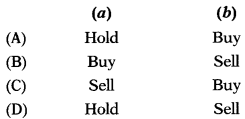(C)
The value of zero coupon bond is the present value of ₹ 2,50,000 for 5 years discounted at 8%.
The value of zero coupon bond = 2,50,000 × 0.681 = ₹ 1,70,250
Investment Decisions:
(a) If the current market price of ZCB is ₹ 1,85,000, investor should sell the bond as market price is more than fair market value of the bond.
(b) If the current market price of ZCB is ₹ 1,65,000, investor should buy the bond as market price is less than fair market value of the bond.

Question 61.
Agfa Industries is planning to issue a debenture series on the following terms: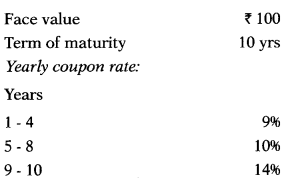Current market rate on similar debentures is 15% p.a. The Company proposes to price the issue in such a manner that it can yield 16% compounded rate of return to the investors. Debentures will be redeemed at 5% premium on maturity. Determine the issue price of the debentures.
(A) ₹ 71.33
(B) ₹ 79.42
(C) ₹ 83.67
(D) ₹ 70.44
(A) ₹ 71.33

Question 62.
John holds 10 Bonds whose face value is ₹ 1,000. Coupon rate is 9%. Yield is 12% and bond matures in 3 years. What is the value of John’s total investment today?
(A) ₹ 10,000
(B) ₹ 9,282
(C) ₹ 10,822
(D) ₹ 9,984
(B) ₹ 9,282Question 63.
Parag holds 10 Bonds whose face value is ₹ 1,000. Coupon rate is 10%. Yield is 12% and bond matures in 5 years. What is the value of Parag’s total investment today?
(A) ₹ 10,000
(B) ₹ 9,275
(C) ₹ 10,527
(D) ₹ 9,875
(B) ₹ 9,275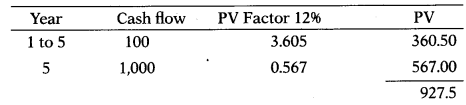Value 10 bonds = 927.5 × 10 9,275

Question 64.
Ram holds 100 irredeemable preference shares of Modi Ltd. coupon rate is 11%. Yield is 13%. Face value is ₹ 100. What is the value of Ram’s total investment today?
(A) ₹ 8,578
(B) ₹ 8,246
(C) ₹ 8,462
(D) ₹ 9,578
(C) ₹ 8,462$$\frac{11}{0.13}=84.62$$
Value of I 00 preference shares = 100 × 84.62 = 8,462

Question 65.
Lakhan holds 100 irredeemable preference shares of Maya Ltd. Coupon rate is 12%. Yield is 13%. Face value is ₹ 100. What is the value of Lakhan’s total investment today?
(A) ₹ 9,438
(B) ₹ 9,231
(C) ₹ 9,134
(D) ₹ 9,292
$$\frac{12}{0.13}$$=92.31